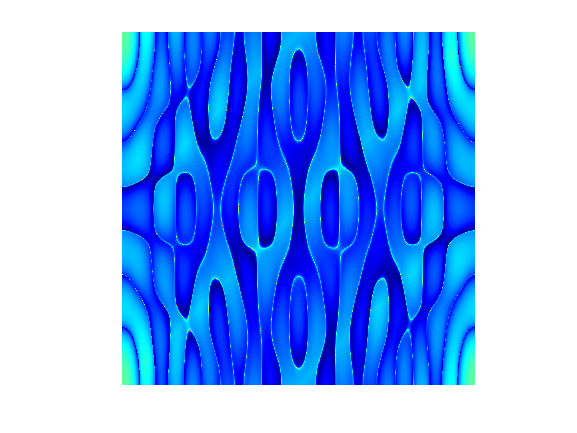# Pattern9Shanshan Wang on 22 Oct 2022
• 6
• 23
• 0
• 1
• 171
n = 2^10;
M = zeros(n);
I = 1:n;
x = I-n/2;
y = n/2-I;
[X,Y] = meshgrid(x,y);
R = 5;
A = (X.^2+Y.^4 <= R^3);
M(A) = 1;
DP = fft2(fftshift(M));
imagesc(abs(log2(DP)))
colormap jet
axis image off##### Remix Tree
+ 1 more
•••